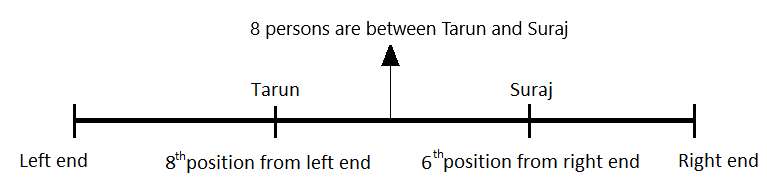Question of The Day21-07-2022

In a row of students, Tarun is standing at the 8th position from the left end of the row and Suraj is standing at the 6th position from the right end of the row. There are 8 students standing between Tarun and Suraj. So, what is the total number of students in the row?

Correct Answer : c ) 22

Explanation :

According to the question,

Tarun is standing at 8th position from the left end. This means there are 7 students present on the left side of Tarun.

Similarly, Suraj is present in the 6th position from the right end. This means there are 5 students present on the right side of Suraj.

There are 8 students are standing between Tarun and Suraj.

Therefore, total number of students in the row = 8 + 8 + 6 = 22 studentsHence, c is the correct answer.0